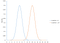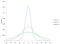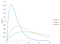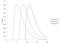Data Distribution and its Parameters

As an ML engineer it is important to know the science of statistics to treat any data in the world and make it talk the way you want to 😎.

The basics start from the Data ,it’s distributions and its parameters.Well you might know about the data and its importance, but today lets see what are these distribution parameters which are also more important.

Data Distribution??

A distribution is simply a collection of data, or scores, on a variable. Usually, these scores are arranged in order from smallest to largest and then they can be presented graphically. (Page 6, Statistics in Plain English, Third Edition, 2010.)

In a practical perspective, we can think of a distribution as a function that describes the relationship between in a sample space.

Density Functions:Distributions are often described in terms of density or density functions. Density functions are functions that describe how the proportion of data or likelihood of the proportion of observations change over the range of the distribution.These are broadly divided into two types -Continuous and discrete

Continuous Distributions can be divided further into PDF and CDF

PDF-Probability Density Functions

Calculates the probability of observing a given value.

Can be used to calculate the likelihood of a given observation in a distribution.

It can also be used to summarise the likelihood of observations across the distribution’s sample space

Plots of the PDF show the familiar shape of a distribution, such as the bell-curve for the Gaussian distribution.

CDF-Cumulative distribution functions

Calculates the probability of an observation equal or less than a value

Rather than calculating the likelihood of a given observation as with the PDF, the CDF calculates the cumulative likelihood for the observation and all prior observations in the sample space.

It allows you to quickly understand and comment on how much of the distribution lies before and after a given value.

A CDF is often plotted as a curve from 0 to 1 for the distribution

Discrete Distributions can be divided into PMF and CDF

PMF-Probability Mass Functions

Characterises the distribution of a discrete random variable.

Same as PDF but on a discrete variable

CDF-Cumulative distribution functions

This is same as that of the continuous but only thing is here it is a discrete variable.

Application of Probability Distribution Functions and Cumulative Distribution Functions

1. To calculate confidence intervals for parameters(we will see this below)and to calculate critical regions for hypothesis tests.
2. For uni variate data, it is often useful to determine a reasonable distributional model for the data.
3. Identifying the exact distribution type will help us to decide which further statistical or Machine learning algorithms can be implemented::- .Statistical intervals and hypothesis tests are often based on specific distributional assumptions. Before computing an interval or test based on a distributional assumption, we need to verify that the assumption is justified for the given data set. In this case, the distribution does not need to be the best-fitting distribution for the data, but an adequate enough model so that the statistical technique yields valid conclusions
4. By assuming a random variable follows an established probability distribution, we can use its derived pmf/pdf and established principles to answer questions we have about the data.

Distribution Parameters(Parameters)

How do you determine the best distribution for a data set or a variable?

The distribution’s parameters define the distribution.

Statistical techniques are used to estimate the parameters of the various distributions.

There are four parameters primarily used in Distribution fitting.Distribution fitting involves estimating the parameters that define the various distributions.

• Location(mean,mode,median):The location parameter of a distribution indicates where the distribution lies along the x-axis (the horizontal axis).
• Scale(standard deviation):The scale parameter of a distribution determines how much spread there is in the distribution
• Shape:The shape parameter of a distribution allows the distribution to take different shapes.
• Threshold:The threshold parameter of a distribution defines the minimum value of the distribution along the x-axis.

Not all parameters exist for each distribution : For example, the normal distribution has only two parameters: location (the average) and scale (the standard deviation). These two parameters completely define the normal distribution.Figure 1: Normal Distribution with Different Locations

The location parameter of a distribution indicates where the distribution lies along the x-axis (the horizontal axis). Figure 1 shows two normal distributions. The location values are different. The blue distribution has a location of 5. The orange distribution has a location of 10. Both have the same standard deviation (or scale in parameter terms).Figure 2: Logistic Distribution with Different Scale Parameters

The scale parameter of a distribution determines how much spread there is in the distribution. The larger the scale parameter, the more spread there is in the distribution. and vice versa . Figure 2 shows the logistic distribution with three different scale parameters: 2(0 to -10=2), 5(0 to -25), and 8(0 to 40). The location for all three curves is 0.Figure 3: Gamma Distribution with Different Shape Parameters

The shape parameter of a distribution allows the distribution to take different shapes.The two distributions above, the normal and the logistic distributions, do not have a shape parameter.The larger the shape parameter, the more the distribution tends to be skewed o the left.The smaller the shape parameter, the more the distribution tends to be skewed to the right

Figure 3 shows how changing the shape parameter impacts the gamma distribution. The scale parameter for the gamma distribution in Figure 3 is 2. The gamma distribution does not have a location parameter.Figure 4: Gamma Distribution with Different Threshold Values

The threshold parameter of a distribution defines the minimum value of the distribution along the x-axis.

The distribution cannot have any values below this threshold.

Figure 4 is the gamma distribution with three different threshold values: 3, 6 and 9. The scale and shape parameter are both 2.

Reference:

Data and Maths Evangelist|| Machine Learning|| Working in Tech Mahindra

## More from Rahul Kaushik

Data and Maths Evangelist|| Machine Learning|| Working in Tech Mahindra